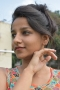## What is 'Space Complexity’?Published on 12-Jul-2018 12:48:25
Space ComplexitySpace complexity is an amount of memory used by the algorithm (including the input values of the algorithm), to execute it completely and produce the result.We know that to execute an algorithm it must be loaded in the main memory. The memory can be used in different forms:Variables (This ... Read More

## Algorithms and ComplexitiesPublished on 12-Jul-2018 12:08:23
AlgorithmAn algorithm is a finite set of instructions, those if followed, accomplishes a particular task. It is not language specific, we can use any language and symbols to represent instructions.The criteria of an algorithmInput: Zero or more inputs are externally supplied to the algorithm.Output: At least one output is produced ... Read More

## Find Weekday using Zeller's AlgorithmPublished on 12-Jul-2018 11:32:47
Zeller’s Algorithm is used to find the weekday from a given date. The formula to find weekday using Zeller’s Algorithm is here:The formula is containing some variables; They are −d − The day of the date.m: It is the month code. From March to December it is 3 to 12, ... Read More

## Parity Check of a NumberPublished on 12-Jul-2018 10:38:39
Parity of a number is based on the number of 1’s present in the binary equivalent of that number. When the count of present 1s is odd, it returns odd parity, for an even number of 1s it returns even parity.As we know that the numbers in computer memory are ... Read More

## Kth Largest Element in an ArrayPublished on 12-Jul-2018 09:23:59
From a set of data, this algorithm will find the largest element to kth largest element of the array.This problem can be solved easily by sorting the array. We can sort them either in ascending order or in descending order. Solving it in descending order, we can get first k ... Read More

## Flood fill AlgorithmPublished on 12-Jul-2018 08:53:11
One matrix is given; the matrix is representing the one screen. Each element (i, j) of the screen is denoted as a pixel, the color of that pixel is marked with different numbers. In this algorithm, the pixels will be filled with new color when it is already in selected ... Read More

## Number to Roman NumeralsPublished on 12-Jul-2018 08:16:50
Roman numbers are non-positional numbers. Some numerals are placed together to form a number in roman numbers. For an example the number 75 can be expressed as 75 = 50 + 10 + 10 + 5, so the roman numerals are LXXV.In this problem one number is provided in a ... Read More

## Check if given four points form a SquarePublished on 12-Jul-2018 07:46:41
In a 2d plane, four points are given. This algorithm will check whether four points are forming a square or not.Checking for a square we have to match these conditions −All four sides formed by given points are same.All two connecting sides are right-angled.Input and OutputInput: Four points {(20, 10), ... Read More

## Factorial of a large numberPublished on 12-Jul-2018 07:23:27
In computers, variables are stored in memory locations. But the size of the memory location is fixed, so when we try to find the factorial of some greater value like 15! or 20! the factorial value exceeds the memory range and returns wrong results.For calculation of large numbers, we have ... Read More

## Find GCD of two numbersPublished on 12-Jul-2018 06:16:45
In mathematics, Greatest Common Divisor (GCD) is the largest possible integer, that divides both of the integers. The condition is that the numbers must be non-zero.We will follow the Euclidean Algorithm to find the GCD of two numbers.Input and OutputInput: Two numbers 51 and 34 Output: The GCD is: 17AlgorithmfindGCD(a, ... Read More